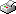Print This Page Daniel Bruce -- doctoral thesis -- Optimal Design and Inference for Correlated Bernoulli Variables using a Simplified Cox Model Abstract This thesis proposes a simplification of the model for dependent Bernoulli variables presented in Cox and Snell (1989). The simplified model, referred to as the simplified Cox model, is developed for identically distributed and dependent Bernoulli variables. Properties of the model are presented, including expressions for the loglikelihood function and the Fisher information. The special case of a bivariate symmetric model is studied in detail. For this particular model, it is found that the number of design points in a locally D-optimal design is determined by the log-odds ratio between the variables. Under mutual independence, both a general expression for the restrictions of the parameters and an analytical expression for locally D-optimal designs are derived. Focusing on the bivariate case, score tests and likelihood ratio tests are derived to test for independence. Numerical illustrations of these test statistics are presented in three examples. In connection to testing for independence, an E-optimal design for maximizing the local asymptotic power of the score test is proposed. The simplified Cox model is applied to a dental data. Based on the estimates of the model, optimal designs are derived. The analysis shows that these optimal designs yield considerably more precise parameter estimates compared to the original design. The original design is also compared against the E-optimal design with respect to the power of the score test. For most alternative hypotheses the E-optimal design provides a larger power compared to the original design. Key words: Cox binary model, correlated binary data, log-odds ratio, D-optimality, E-optimality, power maximization, efficiency ISBN 978-91-7155-657-8